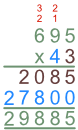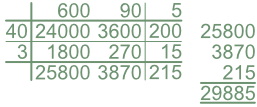Long Multiplication

# Long Multiplication

Basic, GCSE(F),

Long Multiplication involves multiplying two larger numbers together.

This can be carried out by using either the long multiplication method or thebox, or grid, method.  Use whichever method is most familiar to you: both will give the same answer. Other methods also work.

The Long Multiplication method.

Set out the two numbers in rows with the larger number on top.  With the smaller number, select the units digit and carry out a short multiplication on the larger number. Repeat using the tens digit rfom the smaller number on th eline below: as this is a multiple of 10, the short multiplication will be one digit to the left, with the units digit being zero. If necessary, repeat for the hundreds digit, placing zeroes in the units and tens columns.

Finally, add the results of all the multiplications together.

The Grid, or Box, Method.

The Grid Method, or Box Method, works by multiplying every digit of one number against every digit of the other, taking into account the full value of both digits. After the multiplication is complete, the results of each multiplication are added together.

## Examples

1. Multiply 695 x 43 (using the long multiplication method).

Using the Long Multiplication method:695 x 3 = 2085 and
695 x 4 x 10 = 27800 then add 2085 + 27800 = 29885

2. Multiply 695 x 43 (using the box/grid method).